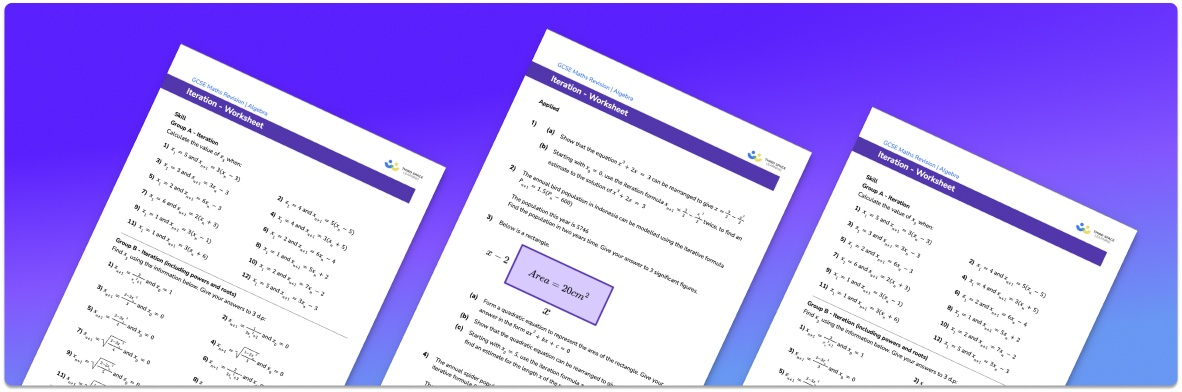# Iteration Worksheet• Section 1 of the iteration worksheet contains 20+ skills-based iteration questions, in 3 groups to support differentiation
• Section 2 contains 3 applied iteration questions with a mix of worded problems and deeper problem solving questions
• Section 3 contains 3 foundation and higher level GCSE exam style questions on iteration
• Answers and a mark scheme for all iteration questions are provided
• Questions follow variation theory with plenty of opportunities for students to work independently at their own level
• All example questions and worksheet problems created by fully qualified expert secondary maths teachers
• Suitable for GCSE maths revision for AQA, OCR and Edexcel exam boards

• This field is for validation purposes and should be left unchanged.

You can unsubscribe at any time (each email we send will contain an easy way to unsubscribe). To find out more about how we use your data, see our privacy policy.

### Iteration at a glance

The iterative process is a method used to find an approximate solution to an equation. The iterative process is most often used for an equation that is not easily solved using other methods such as factorising.

Iteration involves substituting a value into an iteration formula. The output from the formula is then fed back into the formula. This is repeated a certain number of times or until we have found a solution to a particular degree of accuracy, for example a given number of decimal places or significant figures. Each output will be closer than the last to the solution of the equation – the outputs will converge to the solution to the equation.

When using the iterative process, we might be given the iteration formula or we might need to rearrange a given formula into the correct form.

Looking forward, students can then progress to additional solving equations worksheets and other algebra worksheets, for example a sequences worksheet, simultaneous equations worksheet or straight line graphs worksheet.For more teaching and learning support on Algebra our GCSE maths lessons provide step by step support for all GCSE maths concepts.

## Do you have KS4 students who need more focused attention to succeed at GCSE?There will be students in your class who require individual attention to help them succeed in their maths GCSEs. In a class of 30, it’s not always easy to provide.

Help your students feel confident with exam-style questions and the strategies they’ll need to answer them correctly with our dedicated GCSE maths revision programme.

Lessons are selected to provide support where each student needs it most, and specially-trained GCSE maths tutors adapt the pitch and pace of each lesson. This ensures a personalised revision programme that raises grades and boosts confidence.

Find out more